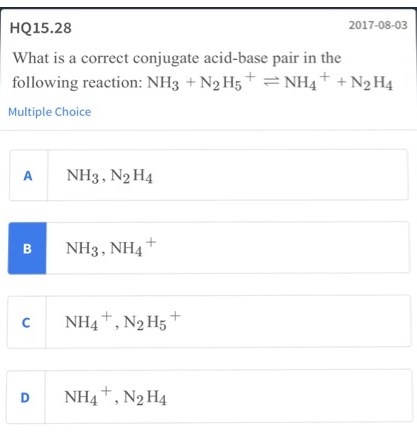# Problem: What is a correct conjugate acid-base pair in the following reaction: NH3 + N2H5+ ⇌ NH4+ + N2H4a. NH3, N2H4b. NH3, NH4+c. NH4+, N2H5+d. NH4+, N2H4

###### FREE Expert Solution
94% (432 ratings)###### Problem Details

What is a correct conjugate acid-base pair in the following reaction: NH3 + N2H5+ ⇌ NH4+ + N2H4

a. NH3, N2H4

b. NH3, NH4+

c. NH4+, N2H5+

d. NH4+, N2H